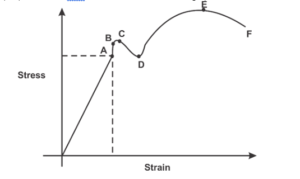Engineering Jobs   »   CIVIL ENGINEERING QUIZ

# DFCCIL’21 CE: Daily Practice Quiz. 31-August-2021

DFCCIL (Dedicated Freight Corridor Corporation of India Limited) Exam Date 2021 on September and October 2021 so boost your preparation by attempt this Civil Engineering MISCELLNEOUS quiz for DFCCIL recruitment 2021.

Quiz: Civil Engineering
Exam: DFCCIL-JE
Topic: MISCELLANEOUS

Each question carries 1 mark
Negative marking: 1/4 mark
Time: 8 Minutes

Q1. Coefficient of friction depends upon
(a) Area of contact only
(b) Nature of surface only
(c) Both (a) and (b)
(d) None of these

Q2. When a road is banked at a curve the angle of banking is determined the relation?
(a) tan θ = v/rg
(b) sin θ = v^2/rg
(c) cos θ = v^2/rg
(d) θ = v^2/rg

Q3. Which of the following is an example of a body undergoing translational equilibrium?
(a) a body at rest on a table
(b) a body travelling in a circular path at a constant speed
(c) a body rotating with constant angular speed about an axis
(d) a body sliding down a frictionless inclined plane

Q4. If two bodies, one light and other heavy, have equal kinetic energy, which one has a greater momentum?
(a) the heavy body
(b) the light body
(c) both have equal momentum
(d) unpredictable

Q5. A thin mild steel wire is loaded by adding loads in equal increments till it breaks. The extension noted with increasing loads will behave as under
(a) uniform throughout
(b) increases uniformly
(c) first increases and then decreases
(d) increases uniformly first and then increases rapidly

Q6. For steel, the ultimate strength in shear as compared to in tension is nearly
(a) same
(b) half
(c) one-third
(d) two-third

SOLUTION

S1. Ans.(b)
Sol. The value of coefficient of friction is vary from 0 to 1 and it only depends upon type of contact surfaces for the smooth surfaces the value of μ = 0

S2. Ans.(a)
Sol. the angle of banking is tan θ = a/g
a for banking curve =ω²r.
a = V²/r² × r = V²/r
tan θ=a/g
∴ tan θ = V²/rg
S3. Ans.(a)
Sol. In case of Translation equillibrium the sum of all the external forces will be zero. So in case a body is rest on the table than all the forces is equal to zero.
S4. Ans.(c)
Sol. Initial momentum = mV1
= 2 × 20 = 40 kgm/sec
Final momentum = mV2 = 2 × (-10) = -20 kg.m/sec
Impulse (change in momentum) = 40 – (-20)
= 60 kg.m/sec
S5. Ans.(d)
Sol. A thin mild steel wire is loaded by adding loads in equal increments till it breaks. The extensions noted with increasing loads will behave as under increases uniformly first and then increases rapidly As, we can seen it in stress-strain mild steel graph.S6. Ans.(d)
Sol. for steel, the ultimate strength in shear as compared to in tension is nearly.
Ultimate strength in shear = 2/3 Ultimate strength in tension.

Sharing is caring!

Thank You, Your details have been submitted we will get back to you.